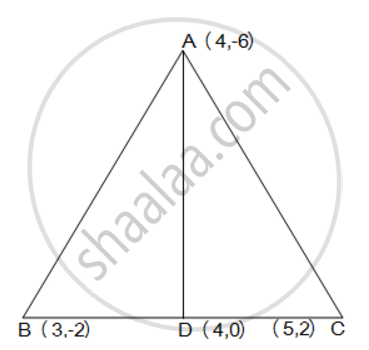# Median of a Triangle Divides It into Two Triangles of Equal Areas. Verify this Result for δAbc Whose Vertices Are a (4, - 6), B (3, - 2) and C (5, 2). - Mathematics

median of a triangle divides it into two triangles of equal areas. Verify this result for ΔABC whose vertices are A (4, - 6), B (3, - 2) and C (5, 2).

#### SolutionLet the vertices of the triangle be A (4, -6), B (3, -2), and C (5, 2).

Let D be the mid-point of side BC of ΔABC. Therefore, AD is the median in ΔABC.

Coordinates of point D = ((3+5)/2, (-2+2)/2) = (4,0)

Area of a triangle =1/2 {x_1 (y_2 - y_3) + x_2 (y_3 - y_1) + x_3 (y_1 - y_2)}

Area of ΔABD =  1/2 [(4) {(-2) - (0)} + 3{(0) - (-6)} + (4) {(-6) - (-2)}]

= 1/2 (-8+18-16)

= -3 square units

However, area cannot be negative. Therefore, area of ΔABD is 3 square units.

Area of ΔABD = 1/2 [(4) {0 - (2)} + 4{(2) - (-6)} + (5) {(-6) - (0)}]

= 1/2 (-8+32-30)

= -3 square units

However, area cannot be negative. Therefore, area of ΔABD is 3 square units.

The area of both sides is same. Thus, median AD has divided ΔABC in two triangles of equal areas

Concept: Area of a Triangle
Is there an error in this question or solution?
Chapter 7: Coordinate Geometry - Exercise 7.3 [Page 170]

#### APPEARS IN

NCERT Class 10 Maths
Chapter 7 Coordinate Geometry
Exercise 7.3 | Q 5 | Page 170
Share